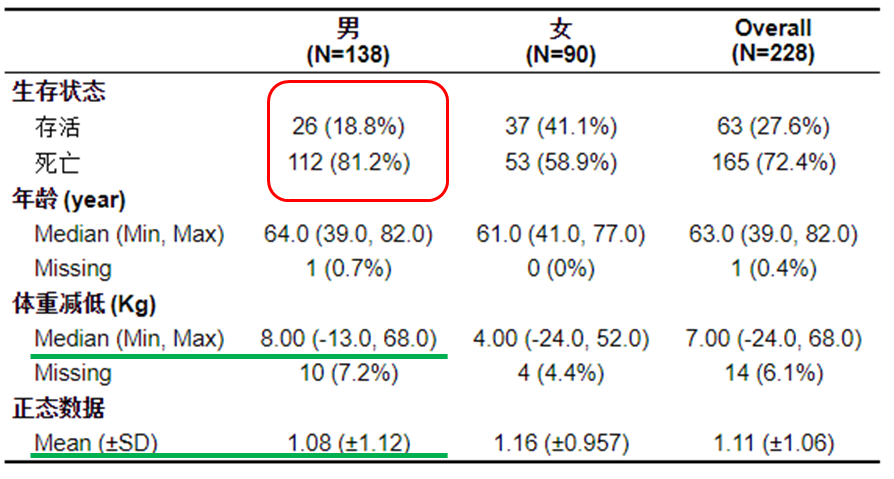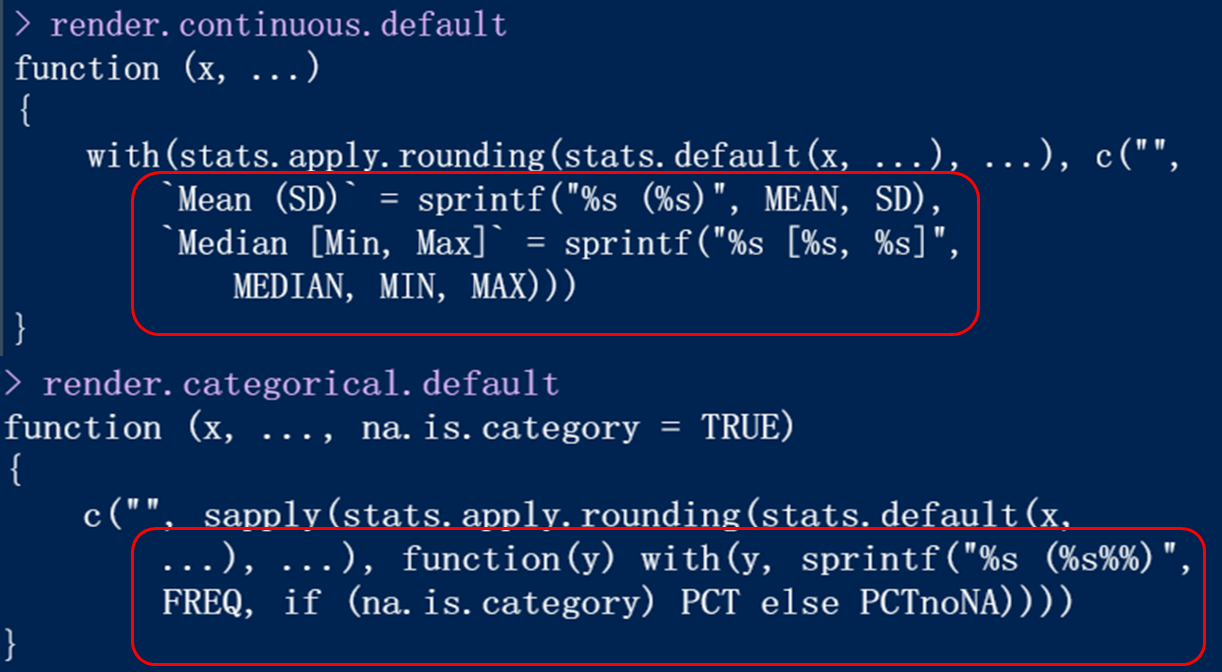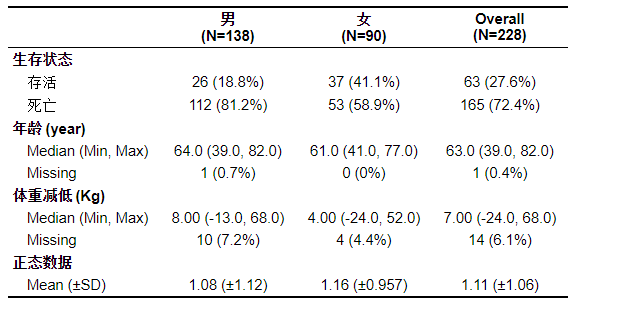# 前言

table1直接画出的三线表对于连续资料是将均值和中位值全部输出的，而不判定数据的正态性。# 二、简单制作

## 1.一般过程（使用formula形式）

rm(list = ls())
library(table1)
library(survival)
options(stringsAsFactors = F)
a <- lung
str(a)

#设置因子。需要注意，分类变量必须设置为因子或者字符串格式，否则它就把它当作数值进行计算了，这里，性别和生存状态都是分类变量。
a$sex <- factor(a$sex,levels = c(1,2),labels = c('男','女'))
a$status <- factor(a$status,levels = c(1,2),labels = c("存活","死亡"))

#可以检验age是非正态的数据，这个p>0.1说明是正态，反之。
shapiro.test(a$age)$p.value

#为了验证能否根据数据的正态性输出不同的格式，这里新增一组正态数据。
a$normdata <- rnorm(nrow(a),1,1) #设置缺失值，看函数是否能识别缺失数据 a$age <- NA

#设置单位，这样可以在行名那儿显示单位，如图中的（years，Kg）
units(a$age) <- 'year' units(a$wt.loss) <- 'Kg'

#设置标签，这里的标签就是行名。否则三线表行名会显示为status，age等等
label(a$status) <- "生存状态" label(a$age) <- "年龄"
label(a$wt.loss) <- "体重减低" label(a$sex) <- "性别"
label(a$normdata) <- "正态数据" table1(~status+age+wt.loss+normdata|sex,data = a)  ## 2.发现问题 运行完代码，确实出现了我们要的三线表，但发现了吗？不论我们的数据是否正态，他都会将均值（标准差）和中位数（极值）两种形式输出。这不是我们想要的。为了去删除，我们还要重新去进行正态性检验然后一行一行删除，显然这不是我们学习R语言的初衷。 ## 3.端倪 抛开这个问题先不谈，我们先学习一下，如何改变这个三线表？ 看一下帮助文档，我已经为大家探索好了，这里表格中的数据是通过render参数传递的。render指向一个render.default函数，render.default中包含了传递连续型变量、分类变量、空值的传递，是函数的形式。调出这两个函数的代码看一下。从函数可以发现分类变量和数值变量的表现形式（红色框标记）。 这里我重写这个render函数和render.continuous.default函数就初步实现函数根据正态检验选择性输出。 代码如下（示例）： 重写render.default函数 rd <- function(x, name, ...){ #这里传入的参数x和name #举个例子，当绘制男性，age这个格子的时候（一下所有的例子都是这个），x就是：数据中性别为男性的所有年龄数据。name就是age，那么这里的a[[name]]用来提取数据a中年龄数据（男性女性都包括） y <- a[[name]] #判断年龄数据中是否存在空值，如果是空值，我们要加入missing行 m = any(is.na(y)) #根据传入的数据是否是数值分别处理 if (is.numeric(y)) { #判断是否正态，并将判断的结果传入my.render.cont函数中 normt=(shapiro.test(y)$p.value>0.1)+2
my.render.cont(x,n=normt,m=m,...)
}else{
#因为分类变量我们不需要处理，这里直接调用默认的函数就行了
render.default(x=x, name=name, ...)
}
}

#重写render.continuous.default函数。
my.render.cont <- function (x,n,m, ...) {
#x和上面函数中的意义一样，n是判断是否正态的结果，m是判断是否空值的结果
a=with(stats.apply.rounding(stats.default(x, ...), ...), c("",
Mean (±SD) = sprintf("%s (±%s)", MEAN, SD),
Median (Min, Max) = sprintf("%s (%s, %s)", MEDIAN, MIN, MAX)
))[-n] #根据正态判断结果判定输出均值还是中位数
if (m) {
a <- c(a,with(stats.apply.rounding(stats.default(is.na(x), ...), ...)$Yes, c(Missing = sprintf("%s (%s%%)", FREQ, PCT)))) #这里就是根据是否存在空值输出missing行 } a } ###最后的最后就是运行我们的绘制三线表函数，render参数和render.continuous参数换成我们重写的函数。 table1(~status+age+wt.loss+normdata|sex,data = a,render = rd,render.continuous=my.render.cont)出图，完美，可以发现正态性的数据和非正态性的数据展现形式是不一样的。 ## 4.代码汇总 最后的最后当然是要把代码汇总，方便你们享用啦 rm(list = ls()) library(survival) library(survminer) library(table1) options(stringsAsFactors = F) #重写函数 rd <- function(x, name, ...){ y <- a[[name]] m = any(is.na(y)) if (is.numeric(y)) { normt=(shapiro.test(y)$p.value>0.1)+2
my.render.cont(x,n=normt,m=m,...)
}else{
render.default(x=x, name=name, ...)
}
}

#重写连续变量处理函数
my.render.cont <- function (x,n,m, ...) {

a=with(stats.apply.rounding(stats.default(x, ...), ...), c("",
Mean (±SD) = sprintf("%s (±%s)", MEAN, SD),
Median (Min, Max) = sprintf("%s (%s, %s)", MEDIAN, MIN, MAX)
))[-n]
if (m) {
a <- c(a,with(stats.apply.rounding(stats.default(is.na(x), ...), ...)$Yes, c(Missing = sprintf("%s (%s%%)", FREQ, PCT)))) } a } #########################分界线############################################# ####上面的部分是可以重复使用的不用变，以下部分需要你换成自己的数据并更改部分内容 ############################################################################ a <- lung str(a) #设置因子、以年龄、性别、体重减低、状态为例子 a$sex <- factor(a$sex,levels = c(1,2),labels = c('男','女')) a$status <- factor(a$status,levels = c(1,2),labels = c("存活","死亡")) a$normdata <- rnorm(nrow(a),1,1)
a$age <- NA #设置单位 units(a$age) <- 'year'
units(a$wt.loss) <- 'Kg' #设置标签 label(a$status) <- "生存状态"
label(a$age) <- "年龄" label(a$wt.loss) <- "体重减低"
label(a$sex) <- "性别" label(a$normdata) <- "正态数据"
table1(~status+age+wt.loss+normdata|sex,data = a)
table1(~status+age+wt.loss+normdata|sex,data = a,render = rd,render.continuous=my.render.cont)
##两相对比，你更喜欢哪一个呢？



# 总结

03-24185
01-092447
11-21498
02-092717
04-05621
01-103206
01-032672
03-201228
10-152395
02-191748
10-241万+
05-021081
01-04988
09-2926
01-203446
02-111207
09-232885
03-24387
11-221558

### “相关推荐”对你有帮助么？

•非常没帮助
•没帮助
•一般
•有帮助
•非常有帮助被折叠的  条评论 为什么被折叠?到【灌水乐园】发言¥2 ¥4 ¥6 ¥10 ¥20余额支付 (余额：-- )扫码支付获取中扫码支付点击重新获取扫码支付1.余额是钱包充值的虚拟货币，按照1:1的比例进行支付金额的抵扣。
2.余额无法直接购买下载，可以购买VIP、C币套餐、付费专栏及课程。余额充值